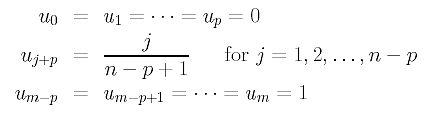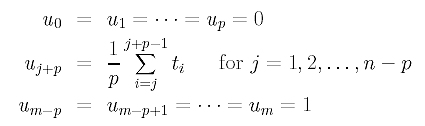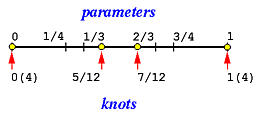# Knot Vector GenerationOnce a set of parameters is obtained, we can generate a knot vector. Suppose we have n+1 parameters t0, t1, ..., tn and degree p. For a B-spline curve of degree p, we need m+1 knots, where m=n+p+1. If the curve is clamped, these knots are u0 = u1 = .... = up = 0, up+1, ..., um-p-1, um-p = um-p+1 = ... = um = 1. More precisely, the first p+1 and last p+1 knots are 0's and 1's, respectively. For the remaining n-p knots, they can be uniformly spaced or chosen properly to achieve some desired conditions.

Suppose the remaining n-p internal knots are uniformly spaced. Then, up = 0, up+1, ..., um-p-1, um-p = 1 divide [0,1] into n-p+1 subintervals. Therefore, the knots areFor example, if we have 6 (n = 5) parameters and the degree is p = 3, we should find (n+p+1)+1 = (5+3+1)+1 = 10 knots (i.e., m=9). Since we use clamped curves, the first and the last four (i.e., p+1) knots should be equal to 0 and 1, respectively. More precisely, we have 0, 0, 0, 0, u4, u5, 1, 1, 1 and 1, and the two internal knots divide [0,1] into three subintervals, each of which has length 1/3. Hence, the knot vector is { 0, 0, 0, 0, 1/3, 2/3, 1, 1, 1, 1}.

A uniformly spaced knot vector does not require the knowledge of the parameters, and is very simple to generate. However, this method is not recommended because, if it is used with the chord length method for global interpolation, the system of linear equations would be singular.

Another popular method for generating knot vector, suggested by de Boor, is to "average" the parameters. Here is the computation formula:Thus, the first internal knot is the average of p parameters t1, t2, ..., tp; the second internal knot is the average of the next p parameters, t2, t3, ..., tp+1. Suppose the 6 (n = 5) parameters are

 t0 t1 t2 t3 t4 t5 0 1/4 1/3 2/3 3/4 1

Suppose we need to generate a vector for degree p = 3. As mentioned earlier, 10 knots (m = 9) are required. Of these 10, the first four and last four knots are 0's and 1's, respectively. Thus, the first internal knot is the average of parameters 1/4, 1/3 and 2/3, and the second internal knot is the average of the next three parameters 1/3, 2/3 and 3/4. The computed knot vector is

 u0 = u1 = u2 = u3 u4 u5 u6 = u7 = u8 = u9 0 (1/4+ 1/3 + 2/3)/3 = 5/12 (1/3 + 2/3 + 3/4)/3 = 7/12 1

The following diagram illustrates the positions of parameters and the generated knots. Note that 0(4) and 1(4) means 0 and 1 are quadruple knots (i.e., multiplicity = 4). As you can see, the first non-zero knot span [0,5/12) contains two parameters, the second non-zero knot span [5/12,7/12) contains no parameter, and the third non-zero knot span [7/12,1) contains two parameters.